# Chunks¶

Dask arrays are composed of many NumPy (or NumPy-like) arrays. How these arrays are arranged can significantly affect performance. For example, for a square array you might arrange your chunks along rows, along columns, or in a more square-like fashion. Different arrangements of NumPy arrays will be faster or slower for different algorithms.

## Specifying Chunk shapes¶

We always specify a `chunks` argument to tell dask.array how to break up the underlying array into chunks. We can specify `chunks` in a variety of ways:

1. A uniform dimension size like `1000`, meaning chunks of size `1000` in each dimension

2. A uniform chunk shape like `(1000, 2000, 3000)`, meaning chunks of size `1000` in the first axis, `2000` in the second axis, and `3000` in the third

3. Fully explicit sizes of all blocks along all dimensions, like `((1000, 1000, 500), (400, 400), (5, 5, 5, 5, 5))`

4. A dictionary specifying chunk size per dimension like ```{0: 1000, 1: 2000, 2: 3000}```. This is just another way of writing the forms 2 and 3 above

Your chunks input will be normalized and stored in the third and most explicit form. Note that `chunks` stands for “chunk shape” rather than “number of chunks”, so specifying `chunks=1` means that you will have many chunks, each with exactly one element.

For performance, a good choice of `chunks` follows the following rules:

1. A chunk should be small enough to fit comfortably in memory. We’ll have many chunks in memory at once

2. A chunk must be large enough so that computations on that chunk take significantly longer than the 1ms overhead per task that Dask scheduling incurs. A task should take longer than 100ms

3. Chunk sizes between 10MB-1GB are common, depending on the availability of RAM and the duration of computations

4. Chunks should align with the computation that you want to do.

For example, if you plan to frequently slice along a particular dimension, then it’s more efficient if your chunks are aligned so that you have to touch fewer chunks. If you want to add two arrays, then its convenient if those arrays have matching chunks patterns

5. Chunks should align with your storage, if applicable.

Array data formats are often chunked as well. When loading or saving data, if is useful to have Dask array chunks that are aligned with the chunking of your storage, often an even multiple times larger in each direction

## Unknown Chunks¶

Some arrays have unknown chunk sizes. This arises whenever the size of an array depends on lazy computations that we haven’t yet performed like the following:

```>>> rng = np.random.default_rng()
>>> x = da.from_array(rng.standard_normal(100), chunks=20)
>>> x += 0.1
>>> y = x[x > 0]  # don't know how many values are greater than 0 ahead of time
```

Operations like the above result in arrays with unknown shapes and unknown chunk sizes. Unknown values within shape or chunks are designated using `np.nan` rather than an integer. These arrays support many (but not all) operations. In particular, operations like slicing are not possible and will result in an error.

```>>> y.shape
(np.nan,)
>>> y
...
ValueError: Array chunk sizes unknown

Summary: to compute chunks sizes, use

```

Using `compute_chunk_sizes()` allows this example run:

```>>> y.compute_chunk_sizes()
>>> y.shape
(44,)
>>> y.compute()
0.78621774046566
```

Note that `compute_chunk_sizes()` immediately performs computation and modifies the array in-place.

Unknown chunksizes also occur when using a Dask DataFrame to create a Dask array:

```>>> ddf = dask.dataframe.from_pandas(...)
dask.array<..., shape=(nan, 2), ..., chunksize=(nan, 2)>
```

Using `to_dask_array()` resolves this issue:

```>>> ddf.to_dask_array(lengths=True)
dask.array<..., shape=(100, 2), ..., chunksize=(20, 2)>
```

More details on `to_dask_array()` are in mentioned in how to create a Dask array from a Dask DataFrame in the documentation on Dask array creation.

## Chunks Examples¶

In this example we show how different inputs for `chunks=` cut up the following array:

```1 2 3 4 5 6
7 8 9 0 1 2
3 4 5 6 7 8
9 0 1 2 3 4
5 6 7 8 9 0
1 2 3 4 5 6
```

Here, we show how different `chunks=` arguments split the array into different blocks

chunks=3: Symmetric blocks of size 3:

```1 2 3  4 5 6
7 8 9  0 1 2
3 4 5  6 7 8

9 0 1  2 3 4
5 6 7  8 9 0
1 2 3  4 5 6
```

chunks=2: Symmetric blocks of size 2:

```1 2  3 4  5 6
7 8  9 0  1 2

3 4  5 6  7 8
9 0  1 2  3 4

5 6  7 8  9 0
1 2  3 4  5 6
```

chunks=(3, 2): Asymmetric but repeated blocks of size `(3, 2)`:

```1 2  3 4  5 6
7 8  9 0  1 2
3 4  5 6  7 8

9 0  1 2  3 4
5 6  7 8  9 0
1 2  3 4  5 6
```

chunks=(1, 6): Asymmetric but repeated blocks of size `(1, 6)`:

```1 2 3 4 5 6

7 8 9 0 1 2

3 4 5 6 7 8

9 0 1 2 3 4

5 6 7 8 9 0

1 2 3 4 5 6
```

chunks=((2, 4), (3, 3)): Asymmetric and non-repeated blocks:

```1 2 3  4 5 6
7 8 9  0 1 2

3 4 5  6 7 8
9 0 1  2 3 4
5 6 7  8 9 0
1 2 3  4 5 6
```

chunks=((2, 2, 1, 1), (3, 2, 1)): Asymmetric and non-repeated blocks:

```1 2 3  4 5  6
7 8 9  0 1  2

3 4 5  6 7  8
9 0 1  2 3  4

5 6 7  8 9  0

1 2 3  4 5  6
```

Discussion

The latter examples are rarely provided by users on original data but arise from complex slicing and broadcasting operations. Generally people use the simplest form until they need more complex forms. The choice of chunks should align with the computations you want to do.

For example, if you plan to take out thin slices along the first dimension, then you might want to make that dimension skinnier than the others. If you plan to do linear algebra, then you might want more symmetric blocks.

Modern NDArray storage formats like HDF5, NetCDF, TIFF, and Zarr, allow arrays to be stored in chunks or tiles so that blocks of data can be pulled out efficiently without having to seek through a linear data stream. It is best to align the chunks of your Dask array with the chunks of your underlying data store.

However, data stores often chunk more finely than is ideal for Dask array, so it is common to choose a chunking that is a multiple of your storage chunk size, otherwise you might incur high overhead.

For example, if you are loading a data store that is chunked in blocks of `(100, 100)`, then you might choose a chunking more like `(1000, 2000)` that is larger, but still evenly divisible by `(100, 100)`. Data storage technologies will be able to tell you how their data is chunked.

## Rechunking¶

 `rechunk`(x[, chunks, threshold, ...]) Convert blocks in dask array x for new chunks.

Sometimes you need to change the chunking layout of your data. For example, perhaps it comes to you chunked row-wise, but you need to do an operation that is much faster if done across columns. You can change the chunking with the `rechunk` method.

```x = x.rechunk((50, 1000))
```

Rechunking across axes can be expensive and incur a lot of communication, but Dask array has fairly efficient algorithms to accomplish this.

Note: The rechunk method expects the output array to have the same shape as the input array and does not support reshaping. It is important to ensure that the desired output shape matches the input shape before using rechunk.

You can pass rechunk any valid chunking form:

```x = x.rechunk(1000)
x = x.rechunk((50, 1000))
x = x.rechunk({0: 50, 1: 1000})
```

## Reshaping¶

The efficiency of `dask.array.reshape()` can depend strongly on the chunking of the input array. In reshaping operations, there’s the concept of “fast-moving” or “high” axes. For a 2d array the second axis (`axis=1`) is the fastest-moving, followed by the first. This means that if we draw a line indicating how values are filled, we move across the “columns” first (along `axis=1`), and then down to the next row. Consider `np.ones((3, 4)).reshape(12)`: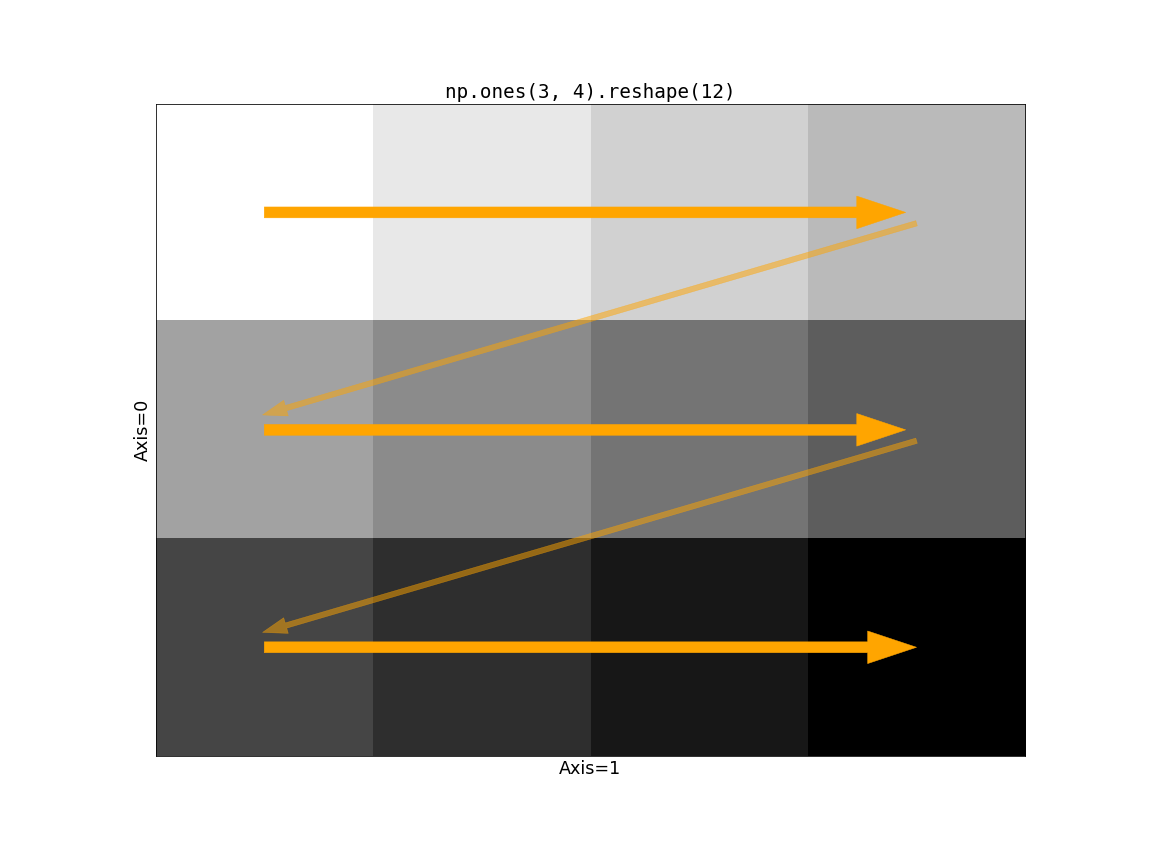Now consider the impact of Dask’s chunking on this operation. If the slow-moving axis (just `axis=0` in this case) has chunks larger than size 1, we run into a problem.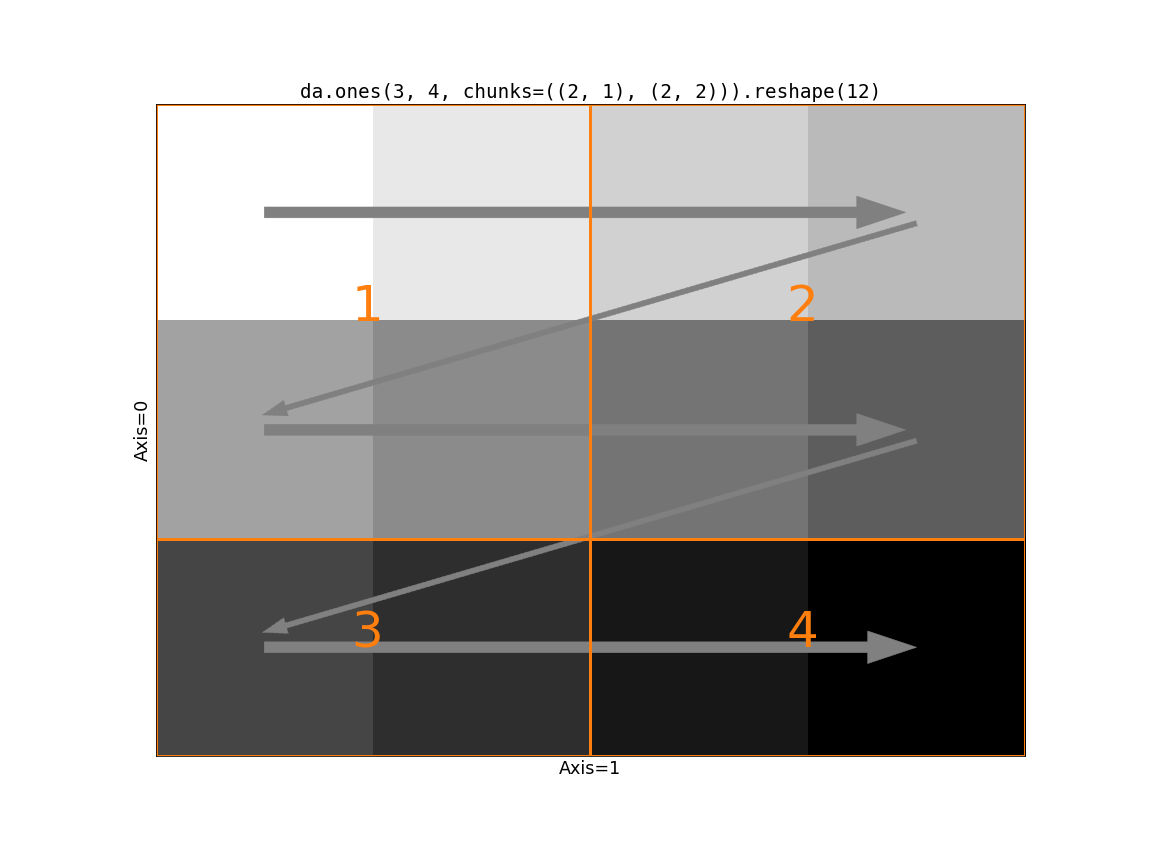The first block has a shape `(2, 2)`. Following the rules of `reshape` we take the two values from the first row of block 1. But then we cross a chunk boundary (from 1 to 2) while we still have two “unused” values in the first block. There’s no way to line up the input blocks with the output shape. We need to somehow rechunk the input to be compatible with the output shape. We have two options

1. Merge chunks using the logic in `dask.array.rechunk()`. This avoids making two many tasks / blocks, at the cost of some communication and larger intermediates. This is the default behavior.

2. Use `da.reshape(x, shape, merge_chunks=False)` to avoid merging chunks by splitting the input. In particular, we can rechunk all the slow-moving axes to have a chunksize of 1. This avoids communication and moving around large amounts of data, at the cost of a larger task graph (potentially much larger, since the number of chunks on the slow-moving axes will equal the length of those axes.).

Visually, here’s the second option: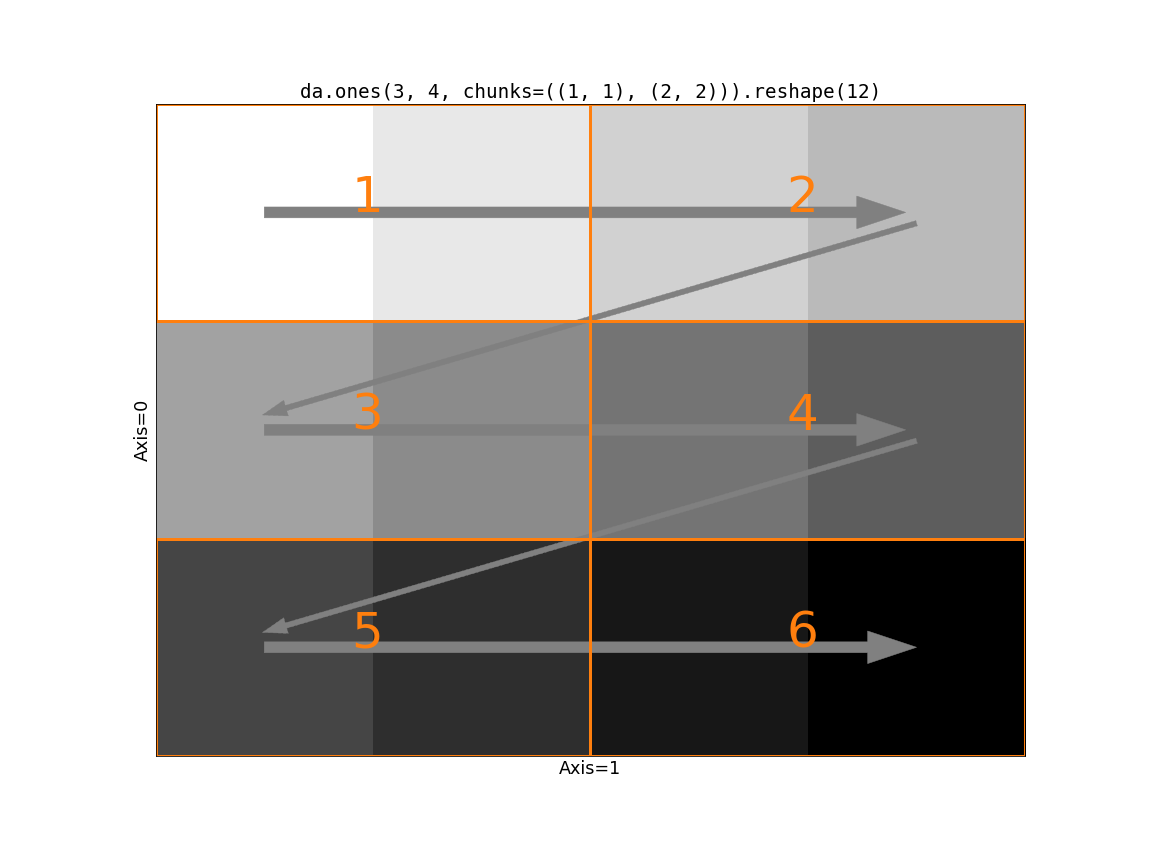Which if these is better depends on your problem. If communication is very expensive and your data is relatively small along the slow-moving axes, then `merge_chunks=False` may be better. Let’s compare the task graphs of these two on a problem reshaping a 3-d array to a 2-d, where the input array doesn’t have `chunksize=1` on the slow-moving axes.

```>>> a = da.from_array(np.arange(24).reshape(2, 3, 4), chunks=((2,), (2, 1), (2, 2)))
>>> a
dask.array<array, shape=(2, 3, 4), dtype=int64, chunksize=(2, 2, 2), chunktype=numpy.ndarray>
>>> a.reshape(6, 4).visualize()
```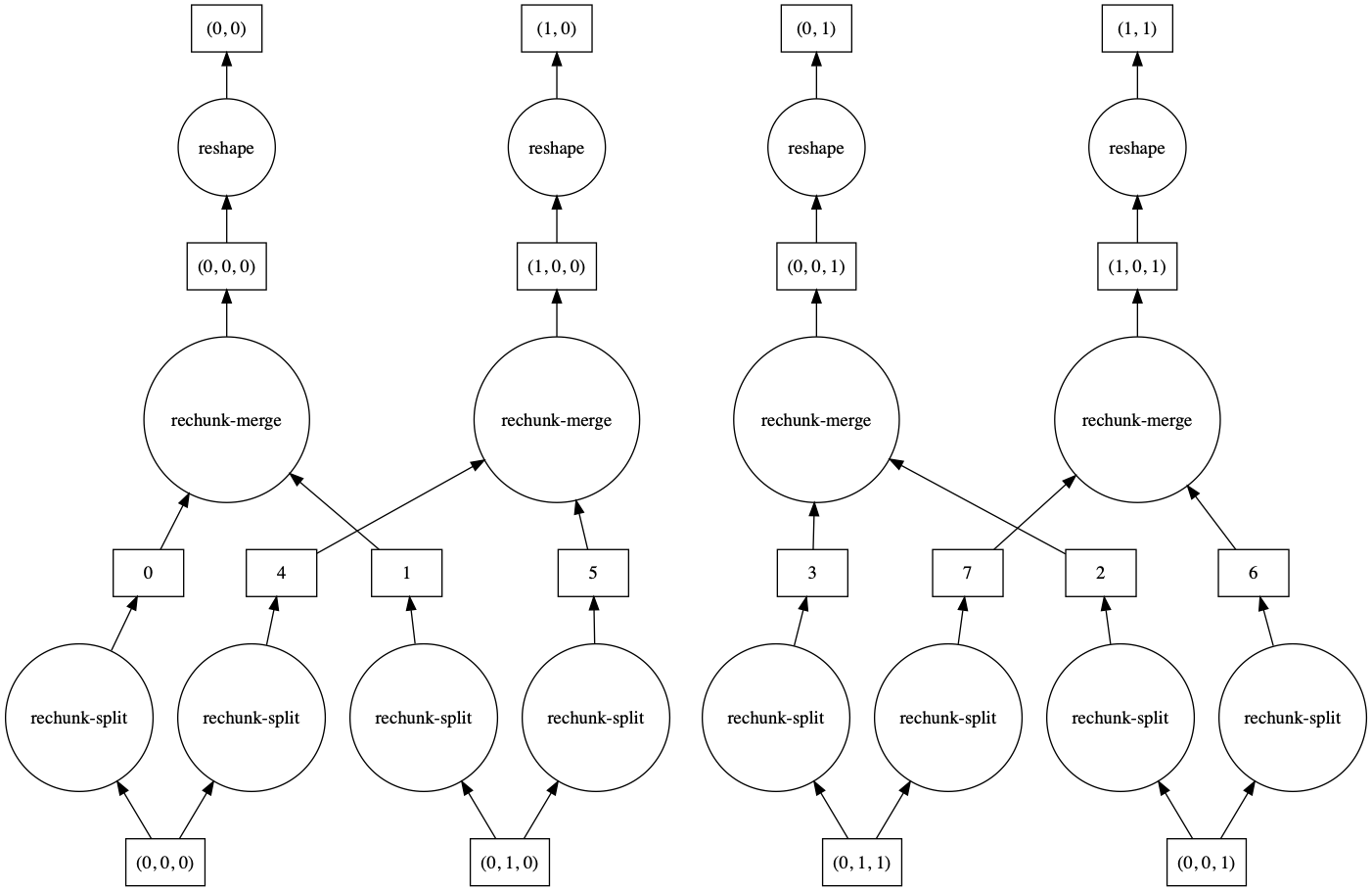```>>> a.reshape(6, 4, merge_chunks=False).visualize()
```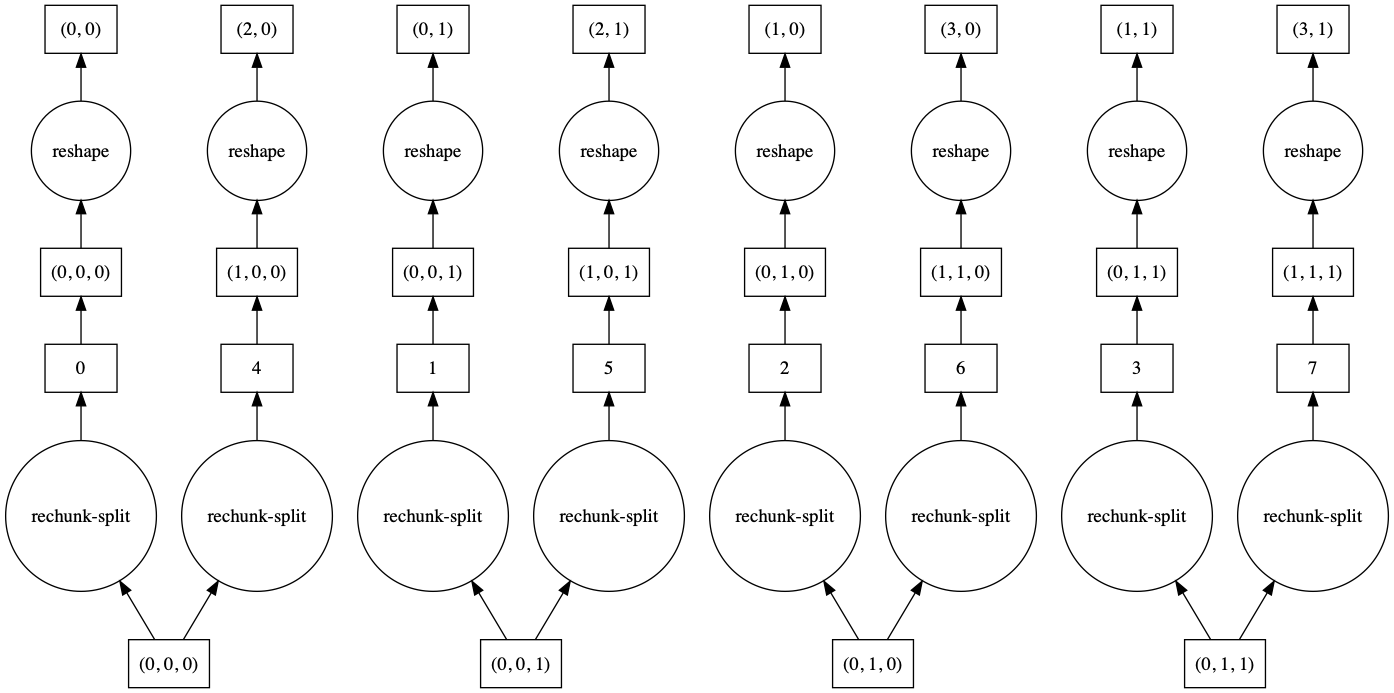By default, some intermediate chunks chunks are merged, leading to a more complicated task graph. With `merge_chunks=False` we split the input chunks (leading to more overall tasks, depending on the size of the array) but avoid later communication.

## Automatic Chunking¶

Chunks also includes three special values:

1. `-1`: no chunking along this dimension

2. `None`: no change to the chunking along this dimension (useful for rechunk)

3. `"auto"`: allow the chunking in this dimension to accommodate ideal chunk sizes

So, for example, one could rechunk a 3D array to have no chunking along the zeroth dimension, but still have sensible chunk sizes as follows:

```x = x.rechunk({0: -1, 1: 'auto', 2: 'auto'})
```

Or one can allow all dimensions to be auto-scaled to get to a good chunk size:

```x = x.rechunk('auto')
```

Automatic chunking expands or contracts all dimensions marked with `"auto"` to try to reach chunk sizes with a number of bytes equal to the config value `array.chunk-size`, which is set to 128MiB by default, but which you can change in your configuration.

```>>> dask.config.get('array.chunk-size')
'128MiB'
```

Automatic rechunking tries to respect the median chunk shape of the auto-rescaled dimensions, but will modify this to accommodate the shape of the full array (can’t have larger chunks than the array itself) and to find chunk shapes that nicely divide the shape.

These values can also be used when creating arrays with operations like `dask.array.ones` or `dask.array.from_array`

```>>> dask.array.ones((10000, 10000), chunks=(-1, 'auto'))
dask.array<wrapped, shape=(10000, 10000), dtype=float64, chunksize=(10000, 1250), chunktype=numpy.ndarray>
```